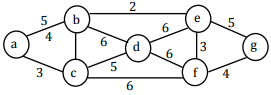# Programming and Data Structures - Online Test

Q1. In which one of the following page replacement policies, Belady’s anomaly may occur?
Explaination / Solution:
No Explaination.

Q2. What is the number of swaps required to sort n elements using selection sort, in the worst case?
Explaination / Solution:
No Explaination.

Q3. The keys 12, 18, 13, 2, 3, 23, 5 and 15 are inserted into an initially empty hash table of length 10 using open addressing with hash function h(k) = k mod 10 and linear probing. What is the resultant hash table?
Explaination / Solution:
No Explaination.

Q4. Consider the following graph:Which of the following is NOT the sequence of edges added to the minimum spanning tree using Kruskal’s algorithm?
Explaination / Solution:
No Explaination.

Q5. The following key values are inserted into a B+-tree in which order of the internal nodes is 3, and that of the leaf nodes is 2, in the sequence given below. The order of internal nodes is the maximum number of tree pointers in each node, and the order of leaf nodes is the maximum number of data items that can be stored in it. The B+-tree is initially empty. 10, 3, 6, 8, 4, 2, 1. The maximum number of times leaf nodes would get split up as a result of these insertions is
Explaination / Solution:
No Explaination.

Q6. Which one of the following array represents a binary max-heap?
Explaination / Solution:
No Explaination.

Q7. A subsequence of a given sequence is just the given sequence with some elements (possibly none or all) left out. We are given two sequences X[m] and Y[n] of lengths m and n, respectively, with indexes of X and Y starting from 0.
We wish to find the length of the longest common subsequence (LCS) of X[m] and Y[n] as l(m, n), where an incomplete recursive definition for the function l(i, j) to compute the length of the LCS of X[m] and Y[n] is given below:
l(i, j) = 0, if either i = 0 or j = 0
= expr1, if i, j>0 and x[i - 1] = Y[j - 1]
= expr2, if i, j>0 and x[i - 1] ≠ Y[j - 1]
Which one of the following option is correct?
Explaination / Solution:
No Explaination.

Q8. A subsequence of a given sequence is just the given sequence with some elements (possibly none or all) left out. We are given two sequences X[m] and Y[n] of lengths m and n, respectively, with indexes of X and Y starting from 0. The values of l(i, j) could be obtained by dynamic programming based on the correct recursive definition of l(i, j) of the form given above, using an array L[M, N], where M = m + 1 and N = n + 1, such that L[i, j] = l(i, j). Which of the following statements would be TRUE regarding the dynamic programming solution for the recursive definition of l(i, j)?
Explaination / Solution:
No Explaination.

Q9. Let G=(V, E) be a graph. Definewhere id is the number of vertices of degree d in G. If S and T are two different trees with ξ(S) = ξ(T), then
Explaination / Solution:
No Explaination.

Q10. In a binary tree with n nodes, every node has an odd number of descendants. Every node is considered to be its own descendant. What is the number of nodes in the tree that have exactly one child?
Explaination / Solution:
No Explaination.